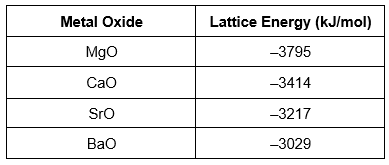Problem: Consider the following trend in the lattice energies of the alkaline earth metal oxides:Explain this trend.

85% (63 ratings)
FREE Expert Solution

We are being asked to explain the following the trend in the lattice energies of the alkaline earth metal oxides:

 Metal Oxide Lattice Energy (kJ/mol) MgO -3795 CaO -3414 SrO -3217 BaO -3029

Recall:

Radius = period (i.e. the row in the periodic table) number

To determine the cation and anion charge, recall that some elements have common charges based on what group they belong to on the periodic table:

85% (63 ratings)
Problem Details
Consider the following trend in the lattice energies of the alkaline earth metal oxides:Explain this trend.

What scientific concept do you need to know in order to solve this problem?

Our tutors have indicated that to solve this problem you will need to apply the Lattice Energy concept. You can view video lessons to learn Lattice Energy Or if you need more Lattice Energy practice, you can also practice Lattice Energy practice problems .

How long does this problem take to solve?

Our expert Chemistry tutor, Rae-Anne took 5 minutes to solve this problem. You can follow their steps in the video explanation above.

What professor is this problem relevant for?

Based on our data, we think this problem is relevant for Professor Couch's class at Sierra College.

What textbook is this problem found in?

Our data indicates that this problem or a close variation was asked in . You can also practice practice problems .